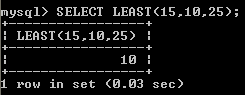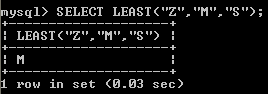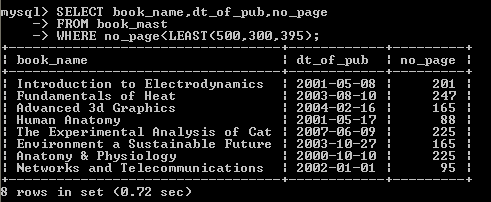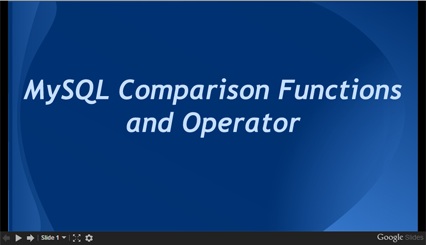# MySQL LEAST() function

## LEAST() function

MySQL LEAST() function returns the smallest argument from two or more arguments.

Syntax:

`LEAST(value1,value2,...)`

The arguments are compared using the following rules:

• If any argument is NULL, the result is NULL. No comparison is needed.
• If the return value is used in an INTEGER context or all arguments are integer-valued, they are compared as integers.
• If the return value is used in a REAL context or all arguments are real-valued, they are compared as reals.
• If the arguments comprise a mix of numbers and strings, they are compared as numbers.
• If any argument is a nonbinary (character) string, the arguments are compared as nonbinary strings.
• In all other cases, the arguments are compared as binary strings.

MySQL Version: 5.6

Example: MySQL LEAST() function

The following MySQL statement will find the smallest out of the list of arguments.

Code:

``````SELECT LEAST(15,10,25);
```
```

Output:Example: MySQL LEAST() function using string

The following MySQL statement will find the smallest out of the list of arguments. It returns M, since S and Z come after M.

Code:

``````SELECT LEAST("Z","M","S");
```
```

Output:Example: MySQL LEAST() function with where clause

The following MySQL statement will fetch those books from book_mast table which have less number of pages than lowest argument returning from the LEAST() function.

Code:

``````SELECT book_name,dt_of_pub,no_page
FROM book_mast
WHERE no_page<LEAST(500,300,395);
```
```

Sample table: book_mast

Output:PHP script:

``````<!doctype html>
<html lang="en">
<meta charset="utf-8">
<meta name="viewport" content="width=device-width, initial-scale=1">
<title>example2-least- php mysql examples | w3resource</title>
<meta name="description" content="example2-least- php mysql examples | w3resource">
<body>
<div class="container">
<div class="row">
<div class="col-md-12">
<h2>List of books whose no. of pages are 300, along with their date of publish and number of pages:</h2>
<table class='table table-bordered'>
<tr>
<th>Book</th><th>Date of publish</th><th>Number of pages</th>
</tr>
<?php
\$hostname="your_hostname";
\$db = "your_dbname";
foreach(\$dbh->query('SELECT book_name,dt_of_pub,no_page
FROM book_mast
WHERE no_page<LEAST(500,300,395)') as \$row) {
echo "<tr>";
echo "<td>" . \$row['book_name'] . "</td>";
echo "<td>" . \$row['dt_of_pub'] . "</td>";
echo "<td>" . \$row['no_page'] . "</td>";
echo "</tr>";
}
?>
</tbody></table>
</div>
</div>
</div>
</body>
</html>
```
```

View the example in browser

JSP script:

``````<%@page contentType="text/html" pageEncoding="UTF-8"%>
<%@ page import="java.sql.*" %>
<%@ page import="java.io.*" %>
<!DOCTYPE html>
<html>
<meta http-equiv="Content-Type" content="text/html; charset=UTF-8">
<title>example2-least</title>
<body>
<%
try {
Class.forName("com.mysql.jdbc.Driver").newInstance();
String Host = "jdbc:mysql://localhost:3306/w3resour_bookinfo";
Connection connection = null;
Statement statement = null;
ResultSet rs = null;
connection = DriverManager.getConnection(Host, "root", "datasoft123");
statement = connection.createStatement();
String Data ="SELECT book_name,dt_of_pub,no_page FROM book_mast WHERE no_page<LEAST(500,300,395)";
rs = statement.executeQuery(Data);
%>
<TABLE border="1">
<tr width="10" bgcolor="#9979">
<td>Book</td>
<td>Date of publish</td>
<td>Number of pages</td>
</tr>
<%
while (rs.next()) {
%>
<TR>
<TD><%=rs.getString("book_name")%></TD>
<TD><%=rs.getString("dt_of_pub")%></TD>
<TD><%=rs.getString("no_page")%></TD>
</TR>
<%   }    %>
</table>
<%
rs.close();
statement.close();
connection.close();
} catch (Exception ex) {
out.println("Can’t connect to database.");
}
%>
</body>
</html>
```
```

Online Practice Editor:

Slideshow of MySQL Comparison Function and OperatorsPrevious: ISNULL()
Next: LESS THAN OR EQUAL OPERATOR(<=)

﻿